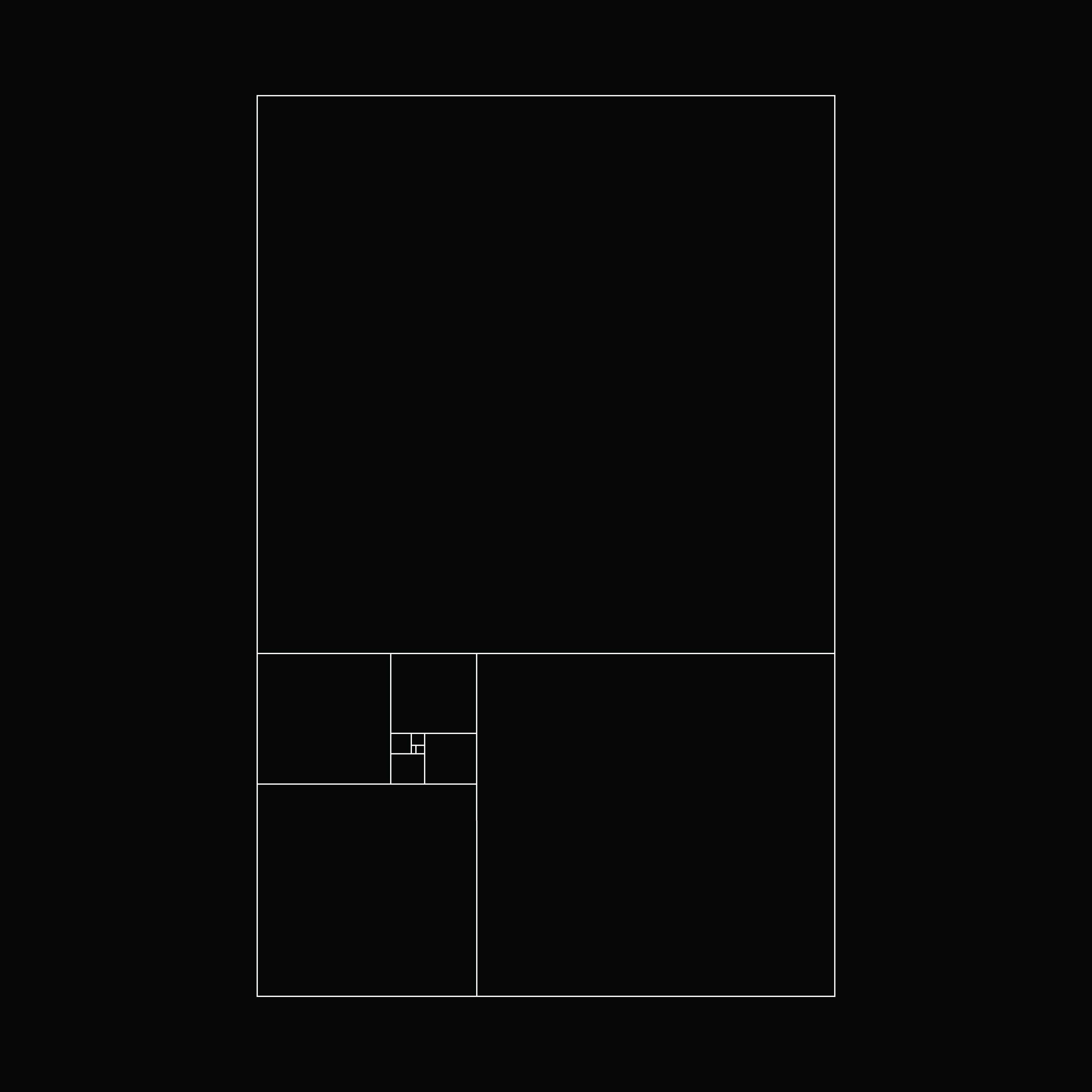## 0,1,1,2,3,5,8,13 . . .

Artwork information
Description

"Fibonacci Sequence"

In mathematics, the Fibonacci numbers, commonly denoted Fn, form a sequence, called the Fibonacci sequence, such that each number is the sum of the two preceding ones, starting from 0 and 1. That is

F0 = 0, F1 = 1

and

Fn = Fn-1 + Fn-2

for n > 1

The beginning of the sequence is thus
0, 1, 1, 2, 3, 5, 8, 13, 21, 34, 55, 89, 144 . . .

Edition of
1
View on Etherscan
View on IPFS